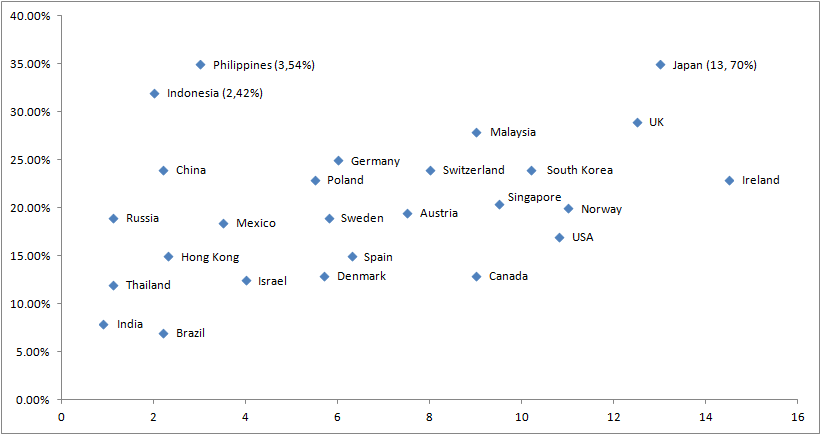### CAT 2008 Question Paper Question 31

Instructions

Directions for the next three questions:

Answer the following questions based on the information given below: Telecom operators get revenue from transfer of data and voice. Average revenue received from transfer of each unit of data is known as ARDT. In the diagram below, the revenue received form data transfer as percentage of total revenue received and the ARDT in US Dollars (USD) are given for various countries.

The X axis represents the ARDT values and the Y axis represents the % of revenue from data transfer as a percentage of the total revenue.Question 31

# If the total revenue received is the same for the pairs of countries listed in the choices below, choose the pair that has approximately the same volume of data transfer.

Solution

We know the following relation that , revenue = volume * ARDT . To have equal volue the ratio of revenue by ARDT should be constant.
Considering UK we have volume = 12.5/30 and of Spain we have 6.5/15 which is fairly constant as compared to others.
Hence option D.